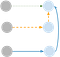# DPState transitions. The first path has 0 extra costs, the rest two paths have an extra cost of 1 for each.

## Code

`int f;const int INF = 0x3f3f3f3f;class Solution {public:    int minSideJumps(vector<int>& b) {        memset(f, 0x3f, sizeof f);        f = 0;        f = f = 1;        const int n = b.size();        for (int i = 1; i < n; ++i) {            for (int j = 0; j < 3; ++j) {                // when obstacle, continue                if (b[i] == j + 1) continue;                for (int k = 0; k < 3; ++k) {                    int cost = 0;                    // if the path contain side jump, set cost to 1.                    if (k != j) cost = 1;                    // if the corresponding path has obstacle, continue                    if (b[i] == k + 1) continue;                    f[i][j] = min(f[i][j], f[i-1][k] + cost);                }            }        }        return *min_element(f[n-1], f[n-1] + 3);    }};`
`Runtime: 288 ms, faster than 80.65% of C++ online submissions for Minimum Sideway Jumps.Memory Usage: 193.9 MB, less than 56.93% of C++ online submissions for Minimum Sideway Jumps.`

# BFS

`const int N = 500010;int visited[N];class Solution {public:    int minSideJumps(vector<int>& b) {        memset(visited, 0, sizeof(visited));        const int n = b.size();        vector<pair<int, int>> this_level;        this_level.emplace_back(0, 1);        visited = 1;        int depth = 0;        while (true) {            int len = this_level.size();            // For each element, see whether can jump to the right as this has 0 cost. If so, put it in the current level.            for (int idx = 0; idx < len; idx++) {                auto [i, j] = this_level[idx];                while (i + 1 < n && !visited[i+1][j] && b[i+1] != j +1) {                    if (i + 1 == n -1) return depth;                    visited[i+1][j] = 1;                    this_level.emplace_back(i+1, j);                    i++;                }            }            vector<pair<int, int>>next_level;            // check whether it can have a side jump. If so, put in next level.            for (auto& [i, j] : this_level) {                for (int k = 0; k < 3; ++k) {                    if (k != j && b[i] != k + 1 && !visited[i][k]){                        visited[i][k] = 1;                        next_level.emplace_back(i, k);                    }                }            }            this_level = next_level;            depth++;        }        return 0;    }};`
`Runtime: 708 ms, faster than 19.90% of C++ online submissions for Minimum Sideway Jumps.Memory Usage: 284 MB, less than 13.61% of C++ online submissions for Minimum Sideway Jumps.`

# Naive Dijkstra by using priority queue

`typedef pair<int, int> PII;const int N = 500010<<2;int dist[N];class Solution {public:    int minSideJumps(vector<int>& b) {        const int n = b.size();        // set dist as INF        memset(dist, 0x3f, sizeof(dist));        // use (i<<2) + j to encode i and j.        dist = 0;        priority_queue<PII, vector<PII>, greater<PII>> pq;        pq.emplace(0, 1);        while (!pq.empty()){            auto [d, u] = pq.top(); pq.pop();            if (d > dist[u]) continue;            auto i = u>>2, j = u&3;            for (int k = 0; k < 3; k++) {                // go right                if (j == k && i + 1 < n && b[i+1] != k + 1) {                    auto v = ((i+1)<<2) + k;                    if (dist[u] >= dist[v]) continue;                    dist[v] = dist[u];                    pq.emplace(dist[v], v);                }                 if (j != k && b[i] != k + 1) {                    auto v = (i<<2) + k;                    if (dist[u] + 1 >= dist[v]) continue;                    dist[v] = dist[u] + 1;                    pq.emplace(dist[v], v);                }            }       }       return min(dist[(n-1)<<2], min(dist[((n-1)<<2) + 1], dist[((n-1)<<2) + 2]));    }};`
`Runtime: 664 ms, faster than 22.43% of C++ online submissions forMinimum Sideway Jumps.Memory Usage: 195.9 MB, less than 54.88% of C++ online submissions for Minimum Sideway Jumps.`

# Improved Dijkstra by using Deque.

## Code

`typedef pair<int, int> PII;const int N = 500010<<2;int dist[N];class Solution {public:    int minSideJumps(vector<int>& b) {        const int n = b.size();        // set dist as INF        memset(dist, 0x3f, sizeof(dist));        // use (i<<2) + j to encode i and j.        dist = 0;        deque<PII> dq;        dq.emplace_front(0, 1);        while (!dq.empty()){            auto [d, u] = dq.front(); dq.pop_front();            if (d > dist[u]) continue;            auto i = u>>2, j = u&3;            for (int k = 0; k < 3; k++) {                // go right                if (j == k && i + 1 < n && b[i+1] != k + 1) {                    auto v = ((i+1)<<2) + k;                    if (dist[u] >= dist[v]) continue;                    dist[v] = dist[u];                    dq.emplace_front(dist[v], v);                }                 if (j != k && b[i] != k + 1) {                    auto v = (i<<2) + k;                    if (dist[u] + 1 >= dist[v]) continue;                    dist[v] = dist[u] + 1;                    dq.emplace_back(dist[v], v);                }            }       }       return min(dist[(n-1)<<2], min(dist[((n-1)<<2) + 1], dist[((n-1)<<2) + 2]));    }};`
`Runtime: 448 ms, faster than 44.93% of C++ online submissions forMinimum Sideway Jumps.Memory Usage: 244 MB, less than 37.14% of C++ online submissions for Minimum Sideway Jumps.`

# Top Down DP

`sys.setrecursionlimit(10**8)class Solution:    def minSideJumps(self, obstacles: List[int]) -> int:        n = len(obstacles)        memo = [[-1]*3 for _ in range(n)]        def dp(i, j):            if memo[i][j] != -1:                return memo[i][j]            if i == n-1:                return 0            if obstacles[i] == j + 1:                return float('inf')            ret = float('inf')            for dj in range(3):                if dj == j and obstacles[i + 1] != dj + 1:                    ret = min(ret, dp(i + 1, dj))                if dj != j and obstacles[i] != dj + 1:                    ret = min(ret, dp(i + 1, dj) + 1)            memo[i][j] = ret            return ret               return dp(0, 1)`

Data Scientist/MLE/SWE @takemobi